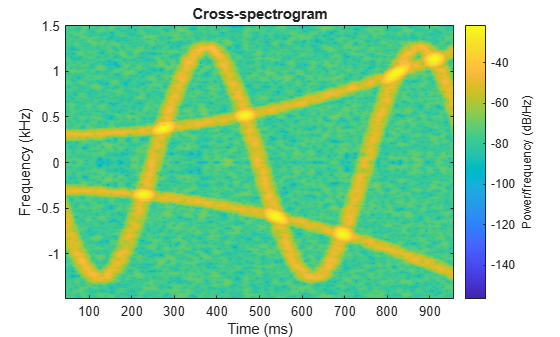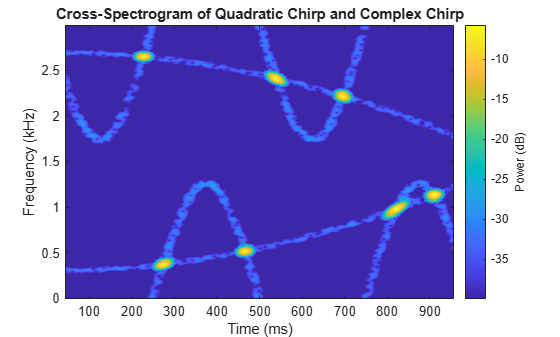Main Content

# Cross-Spectrogram of Complex Signals

Generate two signals, each sampled at 3 kHz for 1 second. The first signal is a quadratic chirp whose frequency increases from 300 Hz to 1300 Hz during the measurement. The chirp is embedded in white Gaussian noise. The second signal, also embedded in white noise, is a chirp with sinusoidally varying frequency content.

```fs = 3000; t = 0:1/fs:1-1/fs; x1 = chirp(t,300,t(end),1300,'quadratic')+randn(size(t))/100; x2 = exp(2j*pi*100*cos(2*pi*2*t))+randn(size(t))/100;```

Compute and plot the cross-spectrogram of the two signals. Divide the signals into 256-sample segments with 255 samples of overlap between adjoining segments. Use a Kaiser window with shape factor β = 30 to window the segments. Use the default number of DFT points. Center the cross-spectrogram at zero frequency.

```nwin = 256; xspectrogram(x1,x2,kaiser(nwin,30),nwin-1,[],fs,'centered','yaxis')```Compute the power spectrum instead of the power spectral density. Set to zero the values smaller than –40 dB. Center the plot at the Nyquist frequency.

```xspectrogram(x1,x2,kaiser(nwin,30),nwin-1,[],fs, ... 'power','MinThreshold',-40,'yaxis') title('Cross-Spectrogram of Quadratic Chirp and Complex Chirp')```The thresholding further highlights the regions of common frequency.

## Support

#### Deep Learning for Signal Processing with MATLAB

Download white paper